Augmented Lyapunov approach to H state estimation of static neural networks with discrete and distributed time-varying delays*
Department of Mathematics, Thiruvalluvar University, Vellore-632115, Tamil Nadu, India

Corresponding author. E-mail: syedgru@gmail.com

*Project supported by the Fund from National Board of Higher Mathematics (NBHM), New Delhi (Grant No. 2/48/10/2011-R&D-II/865).

Abstract

This paper deals with H state estimation problem of neural networks with discrete and distributed time-varying delays. A novel delay-dependent concept of H state estimation is proposed to estimate the H performance and global asymptotic stability of the concerned neural networks. By constructing the Lyapunov–Krasovskii functional and using the linear matrix inequality technique, sufficient conditions for delay-dependent H performances are obtained, which can be easily solved by some standard numerical algorithms. Finally, numerical examples are given to illustrate the usefulness and effectiveness of the proposed theoretical results.

Keyword: 02.30.Hq; 02.30.Ks; 05.45.–a; 02.10.Yn; distributed delay; H state estimation; neural networks; stability analysis
1. Introduction

In the past decades, many kinds of neural networks, such as cellular neural networks, Hopfield neural networks, Cohen– Grossberg neural networks, recurrent neural networks (RNNs), complex dynamical networks (CDNs), bidirectional associative memory (BAM) neural networks, chaotic neural networks (CNNs), and static neural networks (SNNs), have been studied because they have extensive applications in different fields such as fault diagnosis, pattern recognition, signal processing, and parallel computation. Some of these applications require the equilibrium points of the designed networks to be stable. Since axonal signal transmission delays often occur in various neural networks, and may also cause undesirable dynamic network behaviors such as oscillation and instability, it is important to study the stability of the neural networks.

Considerable efforts have been dedicated to the stability analysis of neural networks with delays. Most neural networks can be classified as either continuous[9, 10] or discrete.[11, 12] While, the signal propagation is sometimes instantaneous and can be modeled with discrete delays, it may also be distributed during a certain time period so that distributed delays are incorporated into the model. Therefore, both discrete and time-varying distributed delays should be taken into account when modeling a realistic neural network.

On the other hand, a neural network is a highly interconnected network with a large number of neurons, and as a result most neural networks are large-scale and complex networks. In fact, only partial information about the neuron states is available in the outputs of large-scale neural networks. It is very difficult to obtain the state estimation of the neurons in neural networks because the neural networks have complicated structures. Therefore, it is important to estimate the neuron states through available measurement outputs, and there are many remarkable attempts to design state estimators for various types of neural networks.[16, 17] The delay-dependent H problems for delayed neural networks have received substantial attention from the control community for the past few years.[18, 19] The H control of switched neutral-type neural networks was presented in Ref.   to estimate the robust exponential stability. However, to the best of the authors’ knowledge, the H state estimation of static neural networks with discrete and distributed time-varying delays via augmented Lyapunov approach has not yet been considered, which motivates this study.

In this paper, we study the delay-dependent H state estimation problem for a class of neural networks with discrete and distributed time-varying delays. Our main aim is to design a delay-dependent state estimation gain matrix, such that the concerned system is globally asymptotically stable with a prescribed H performance of disturbance attenuation for all admissible parameters. A sufficient condition for the H state estimation is presented in terms of linear matrix inequality (LMI) using the augmented Lyapunov– Krasovskii functional (LKF) together with the zero function which guarantees the global asymptotic stability of the concerned neural networks. Finally, numerical examples are given to illustrate the usefulness and effectiveness of the proposed method.

The following notations are used. Throughout this paper, ℛ n and ℛ n× n denote, respectively, the n-dimensional Euclidean space and the set of all n × n real matrices. The notation * represents the entries implied by symmetry. The matrix transpose and inverse of A are denoted as AT and A− 1, respectively. The X > 0 means that matrix X is real symmetric positive definite with appropriate dimensions. The I denotes the identity matrix with appropriate dimensions. Let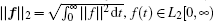, where ‖ f ‖ refers to the Euclidean norm of the functionf(t) at time t, and L2[0, ∞ ) is the space of square integrable vectors on [0, ∞ ).

2. Problem statement

Consider the following neural network with discrete and distributed time-varying delays: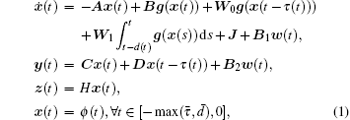where x(t) = [x1(t), x2(t), … , xn(t)]T ∈ ℛ n is the state vector of the network at time t ≥ 0, n is the number of neurons, y(t) ∈ ℛ m is the network measurement, z(t) ∈ ℛ q, to be estimated, is a linear combination of the states, and w(t) ∈ ℛ p is the noise input belonging to L2[0, ∞ ). Here A = diag{a1, … , an} is a diagonal matrix with ai > 0, i = 1, 2, … , n; B, W0, and W1 represent the connection weight matrix, the discretely delayed connection weight matrix, and the distributively delayed connection weight matrix, respectively; and, C, D, B1, B2, and H are known real constant matrices with appropriate dimensions. The g(x(t)) = [g(x1(t)), … , g(xn(t))]T ∈ ℛ n denotes the neuron activation function at time t, and J = [J1, … , Jn]T∈ ℛ n is a constant external input vector. The ϕ (t) is the initial condition. The τ (t) and d(t) denote the discrete time-varying and distributed time-varying delays, respectively, and are assumed to satisfy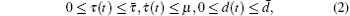where, μ , and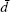are constants.

Assumption 1 Each neuron activation function gi(t), (i = 1, 2, … , n) is continuous and bounded, and satisfies the following condition: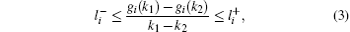with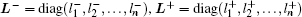are some constants and k1, k2∈ ℛ , k1k2

We consider the following state estimator for the neural network  (1):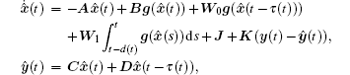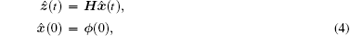where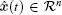denotes the estimated state,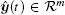,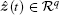denotes the estimated measurements of y(t), z(t), respectively, and K is the estimator gain matrix to be determined.

Define the error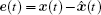) and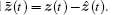. Then, based on Eqs.  (1) and (4), we easily obtain the error system as follows: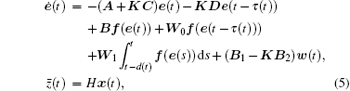where e(t) = [e1(t), e2(t), … , en(t)]T ∈ ℛ n is the state vector of the transformed system and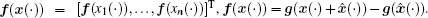. It follows from Assumption 1 that the neural activation function satisfies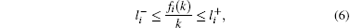where k ∈ ℛ , k ≠ 0.

Definition 1 Given a prescribed level of noise attenuation γ > 0, the error system  (5) is said to be globally asymptotically stable with a prescribed level of noise attenuation γ , if there is a proper state estimator  (4) such that the equilibrium point of the result error system  (5) with w(t) = 0 is globally asymptotically stable, and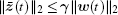under zero-initial conditions for all nonzero w(t) ∈ L2 [0, ∞ ).

Lemma 1 (Schur complement) Let M, P, Q be given matrices such that Q > 0, then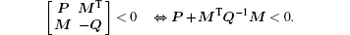Lemma 2 For any constant matrix M ∈ ℛ n× n, M = MT > 0, scalar η > 0, vector function w : [0, η ] → Rn such that the integrations concerned are well defined, we have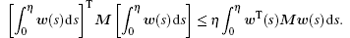Lemma 3 For real matrices P > 0, Mi (i = 1, 2, 3) with appropriate dimensions, and τ (t) satisfying Eq.  (2), we have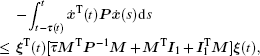where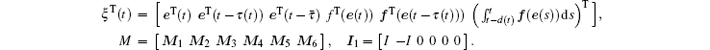Lemma 4 For any scalar τ (t) ≥ 0 and any constant matrix Q ∈ ℜ n× n, Q = QT > 0, the following inequality holds: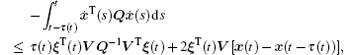where ξ (t) is defined in Lemma 3 and V is a free-weighting matrix with appropriate dimensions.

Lemma 5 The inequalities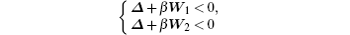are equivalent to the following condition: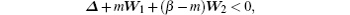where R1, R2, and Δ are constant matrices with appropriate dimensions, variable m ∈ [0, β ] ∈ ℛ , and β > 0.

3. Main results

Theorem 1 For given scalars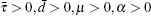and matrix K, the error system  (5) is globally asymptotically stable with H performance γ if there exist real matrices P > 0, P11 > 0, P12, P22 > 0, Q1 > 0, Q2, Q3 > 0, R > 0, S > 0, T1 > 0, T2 > 0, Z1 > 0, Z2, Z3 > 0, T > 0, and any appropriate dimensions matrices Ml, Nl, Um, Vm, (l = 1, 2, … , 6, m = 1, 2), such that the following LMIs hold: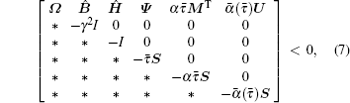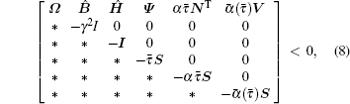where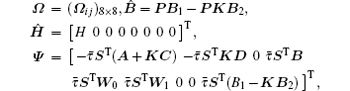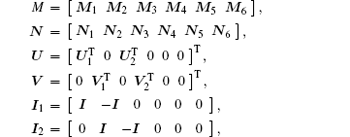with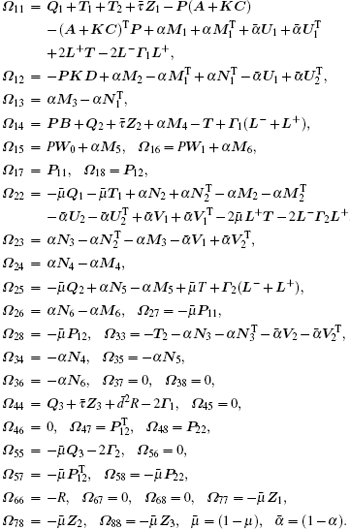Proof Define the Lyapunov functional candidate as follows: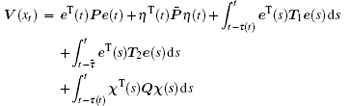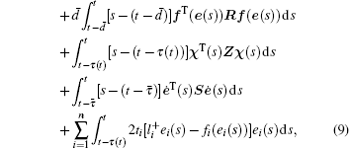where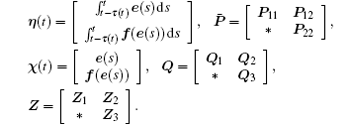By calculating the time derivative of V(xt), we obtain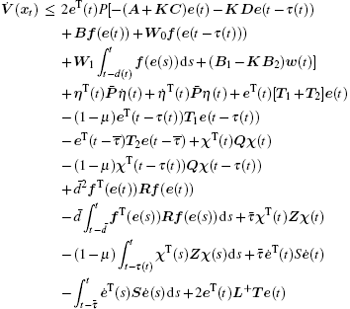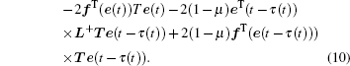By using Lemma 2, we obtain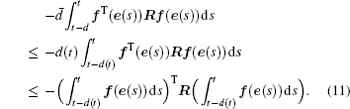From LMI  (10), we havewhere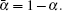By using Lemma 3, we obtain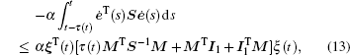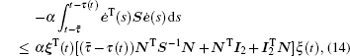where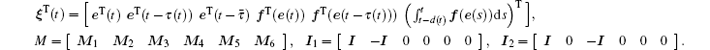By using Lemma 4, we have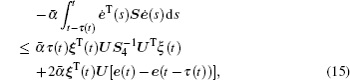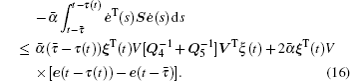In addition, for positive diagonal matrices Γ 1 > 0, Γ 2 > 0, the following equations hold based on formula  (6):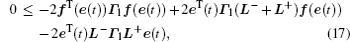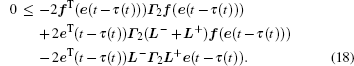By combining inequality  (10) to inequality  (18), we obtain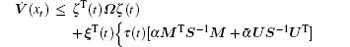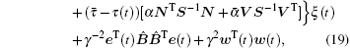where the inequality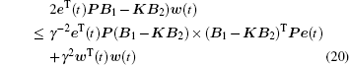is used, ζ (t) is defined by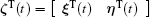and Ω is defined the same as in LMI  (7). Under the zero initial conditions and V(xt)| t= ∞ → 0 for system  (1), we have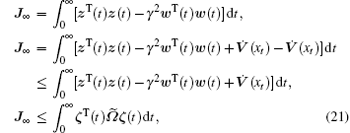where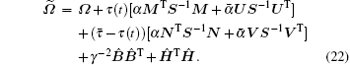Obviously, if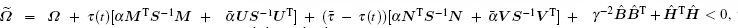then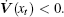Based on Lemma 5, the matrix inequality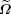is equivalent to the following matrix inequalities: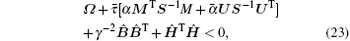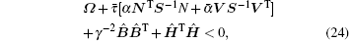when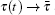and τ (t) → 0, respectively. By applying Schur complement lemma to inequalities  (23) and (24), we obtain LMIs  (7) and (8), respectively. Now it is easy to see that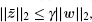for any nonzero w(t) ∈ L2[0, ∞ ) is satisfied. When w(t) ≡ 0, the error system  (5) is globally asymptotically stable.

4. The H performance analysis

In this section, we present a delay-dependent sufficient condition for the solvability of the H performance of the concerned neural network.

Theorem 2 Consider the neural network  (1) and let γ > 0 be a prescribed scalar. The H state estimation problem is solvable if there exist matrices P > 0, P11 > 0, P12, P22 > 0, Q1 > 0, Q2, Q3 > 0, R > 0, S > 0, T1 > 0, T2 > 0, Z1 > 0, Z2, Z3 > 0, T > 0, and any appropriate dimensions matrices Ml, Nl, Um, Vm, (l = 1, 2, … , 6, m = 1, 2), such that the following LMIs hold: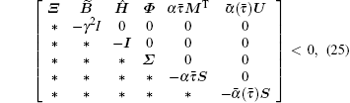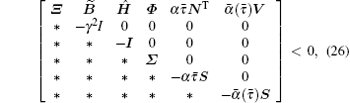where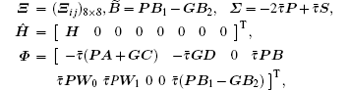with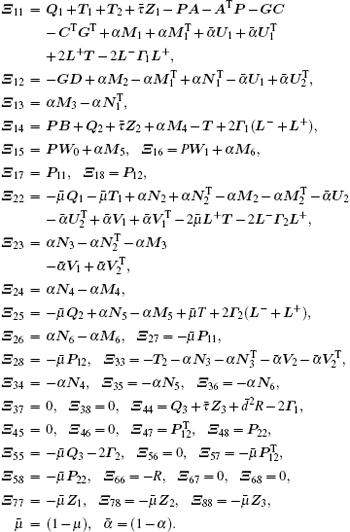Proof Define K = P− 1G, then pre and post multiplying LMIs  (7) and (8) by diag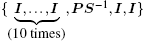and diag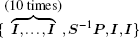respectively. By using the inequality (see Ref.  )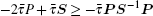we obtain LMIs  (25) and (26). This completes the proof.

Remark 1 In order to show the reduced conservatism of our stability criteria, we consider the following systems as a special case of the system  (1) reduced to a neural network with time-varying delays described by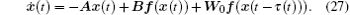Using the same method in Theorem 1, we can obtain the following results.

Corollary 1 For given scalars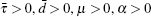and matrix K, the neural network  (27) is globally asymptotically stable with H performance γ if there exist real matrices P > 0, P11 > 0, P12, P22 > 0, Q1 > 0, Q2, Q3 > 0, R > 0, S > 0, T1 > 0, T2 > 0, Z1 > 0, Z2, Z3 > 0, T > 0, and any appropriate dimensions matrices Ml, Nl, Um, Vm, (l = 1, 2, … , 6, m = 1, 2), such that the following LMIs hold: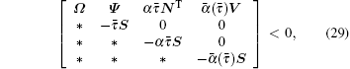where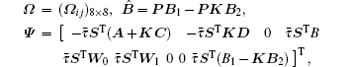with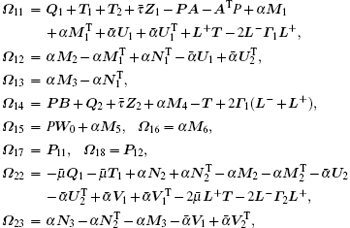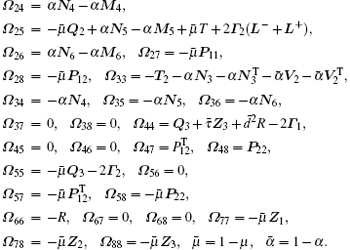Remark 2 Recently, the H state estimation of static neural networks with time-varying delays was studied in Ref.  . Furthermore, the delay-dependent H state estimation of neural networks with discrete and distributed delays was investigated in Ref.  . The H cluster synchronization and state estimation for delayed complex dynamical networks were presented in Ref.  . In Theorem 2, we present a sufficient condition to ensure that the error system  (5) is globally asymptotically stable with an H performance index γ > 0. The delay-dependent condition is derived by constructing an augmented Lyapunov– Krasovskii functional with Lemmas 3 and 4.

5. Numerical examples

Example 1 Consider the neural network  (27) with the following matrix parameters: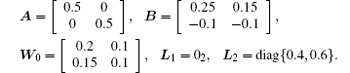By solving LMI in Corollary 1 with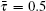, α = 0.5, and μ = 0.3, and using LMI toolbox,  the feasible solutions are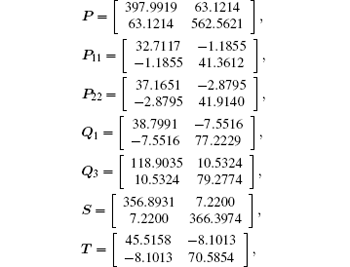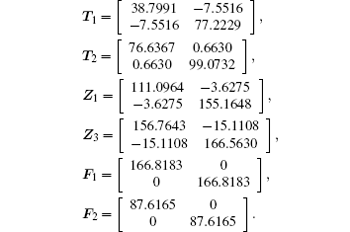Example 2 Consider the neural network  (1) with the following matrix parameters: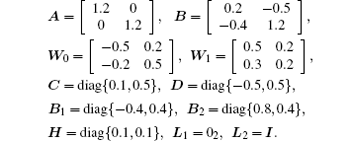By solving LMI in Theorem 2 with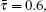d = 0.5, μ = 0.5, and α = 0.3, and using the LMI toolbox, the feasible solutions are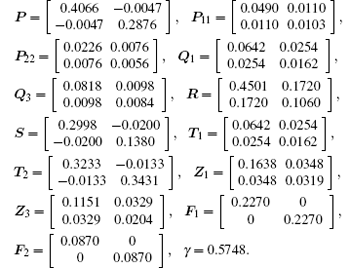The state estimator gain matrix is then obtained as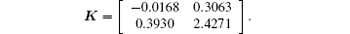The minimum H performance index γ with different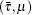and fixed d = 0.5 and μ = 0 is listed in Table  1.Table 1. Minimum H performance index γ with different (, μ ) and fixed d = 0.5 and μ = 0 for Example 2.

Example 3 Consider the neural network  (1) with the following matrix parameters: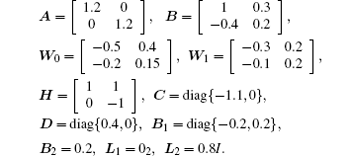Table 2. Minimum H performance index γ with different (,   μ ) and fixed d = 0.5 for Example 3.

By solving LMI in Theorem 2 with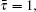, d = 0.5, μ = 0.5, and α = 0.5, and using the LMI toolbox, the state estimator gain matrix is obtained as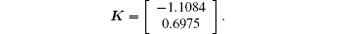The result is presented in Table  2.

6. Conclusion

In this work, we have studied the H state estimation control of neural networks with discrete and distributed time-varying delays. Some improved delay-dependent H state estimation analyses have been established in terms of linear matrix inequality by constructing an appropriate type of LKF for delayed neural networks. It is shown that a desired state estimation controller gain matrix can be constructed when the given linear matrix inequalities are feasible. Numerical examples are given to demonstrate the effectiveness and the usefulness of the proposed method. The results are also compared with existing methods. In this paper, we have considered augmented LKFs to show the less conservative results. However, we construct the augmented LKFs with single integral terms. We would like to point out that it is possible to extend our main results to a more general discrete-time uncertain neutral with interval time-varying and distributed delays by using the augmented LKF approach with triple integral terms. The results will appear in the near future.

Reference
 1 Haykin S 1994 Neural Networks: A Comprehensive Foundation New York Prentice Hall [Cited within:1] 2 Syed Ali M and Balasubramaniam P 2011 Commun. Nonlinear. Sci. Numer. Simulat. 16 2907 DOI:10.1016/j.cnsns.2010.10.011 [Cited within:1] 3 Wang H, Yu Y and Wen G 2014 Neural Netw. 55 98 [Cited within:1] [JCR: 0.362] 4 Syed Ali M 2014 Int. J. Mach. Learn. Cyber. 5 13 DOI:10.1007/s13042-012-0124-6 [Cited within:1] 5 Syed Ali M 2014 Chin. Phys. B 23 060702 DOI:10.1088/1674-1056/23/6/060702 [Cited within:1] [JCR: 1.148] [CJCR: 1.2429] 6 Li H 2014 Neurocomputing 138 78 DOI:10.1016/j.neucom.2014.02.051 [Cited within:1] [JCR: 1.634] 7 Chen Y and Wu Y 2009 Neurocomputing 72 1065 DOI:10.1016/j.neucom.2008.03.006 [Cited within:1] [JCR: 1.634] 8 Syed Ali M 2014 Iranian Journal of Fuzzy Systems 11 1 [Cited within:1] [JCR: 1.056] 9 Syed Ali M and Marudai M 2011 Math. Comput. Modell. 54 1979 DOI:10.1016/j.mcm.2011.05.004 [Cited within:1] [JCR: 1.42] 10 Syed Ali M 2011 Chin. Phys. B 20 080201 DOI:10.1088/1674-1056/20/8/080201 [Cited within:1] [JCR: 1.148] [CJCR: 1.2429] 11 Wu S L, Li K L and Huang T Z 2012 Commun. Nonlinear Sci. Numer. Simulat. 17 3947 DOI:10.1016/j.cnsns.2012.02.013 [Cited within:1] 12 Wang J, Jiang H and Hu C 2014 Neurocomputing 142 542 DOI:10.1016/j.neucom.2014.02.056 [Cited within:1] [JCR: 1.634] 13 Syed Ali M and Saravanakumar R 2014 Chin. Phys. B 23 120201 DOI:10.1088/1674-1056/23/12/120201 [Cited within:1] [JCR: 1.148] [CJCR: 1.2429] 14 Syed Ali M and Saravanakumar R 2014 Appl. Math. Comput. 249 510 DOI:10.1016/j.amc.2014.10.052 [Cited within:1] [JCR: 0.75] 15 Lakshmanan S, Park J H, Jung H Y, Kwon O M and Rakkiyappan R 2013 Neurocomputing 111 81 DOI:10.1016/j.neucom.2012.12.016 [Cited within:1] [JCR: 1.634] 16 Duan Q, Su H and Wu Z G 2012 Neurocomputing 97 16 DOI:10.1016/j.neucom.2012.05.021 [Cited within:1] [JCR: 1.634] 17 Huang H, Huang T and Chen X 2013 IEEE Trans. Circuits Syst. Express Briefs 60 371 DOI:10.1109/TCSII.2013.2258258 [Cited within:1] 18 Phat V N and Trinh H 2013 Neural. Comput. Applic. 22 323 DOI:10.1007/s00521-012-0820-x [Cited within:1] [JCR: 1.168] 19 Huang H and Feng G 2009 IEEE Trans. Circuits Syst. I Reg. Papers 56 846 DOI:10.1109/TCSI.2008.2003372 [Cited within:1] 20 Mathiyalagan K, Sakthivel R and Anthoni S M 2012 Int. J. Adapt. Control Signal Process. 28 429 DOI:10.1002/acs.2332 [Cited within:1] [JCR: 1.219] 21 Boyd B, Ghoui L E, Feron E and Balakrishnan V 1994 Linear Matrix Inequalities in System and Control Theory Philadephia SIAM [Cited within:1] 22 Gu K, Kharitonov V L and Chen J 2003 Stability of Time Delay Systems Boston Birkhuser [Cited within:1] 23 Huang H, Feng G and Cao J 2008 IEEE Trans. Neural Netw. 1329 1329 DOI:10.1109/TNN.2008.2000206 [Cited within:1] 24 Kwon O M, Park J H and Lee S M 2010 J. Optim. Theory Appl. 145 343 DOI:10.1007/s10957-009-9637-x [Cited within:1] 25 Liu Z W and Zhang H G 2010 Acta Automat. Sin. 36 147 DOI:10.1016/S1874-1029(09)60010-0 [Cited within:1] 26 Senthilkumar T and Balasubramaniam P 2011 Appl. Math. Lett. 24 1986 DOI:10.1016/j.aml.2011.05.023 [Cited within:1] [JCR: 1.501] 27 Liu Y, Lee S M, Kwon O M and Park J H 2014 Appl. Math. Comput. 226 589 DOI:10.1016/j.amc.2013.10.075 [Cited within:1] [JCR: 0.75] 28 Qin B and Huang J 2014 Int. J. Math. Comput. Sci. Engg. 8 309 [Cited within:1] 29 Li H 2013 Appl. Math. Modelling 37 7223 DOI:10.1016/j.apm.2013.02.019 [Cited within:1] [JCR: 1.706] 30 Gahinet P, Nemirovski A, Laub A and Chilali M 1995 LMI Control toolbox User’s Guide Natick The Mathworks [Cited within:1]